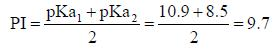Courses

# Heterocyclic Chemistry & Natural Process

## 20 Questions MCQ Test GATE Chemistry Mock Test Series 2020 | Heterocyclic Chemistry & Natural Process

Description
This mock test of Heterocyclic Chemistry & Natural Process for Chemistry helps you for every Chemistry entrance exam. This contains 20 Multiple Choice Questions for Chemistry Heterocyclic Chemistry & Natural Process (mcq) to study with solutions a complete question bank. The solved questions answers in this Heterocyclic Chemistry & Natural Process quiz give you a good mix of easy questions and tough questions. Chemistry students definitely take this Heterocyclic Chemistry & Natural Process exercise for a better result in the exam. You can find other Heterocyclic Chemistry & Natural Process extra questions, long questions & short questions for Chemistry on EduRev as well by searching above.
QUESTION: 1

Solution:
QUESTION: 2

###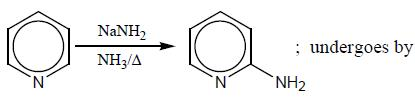Solution: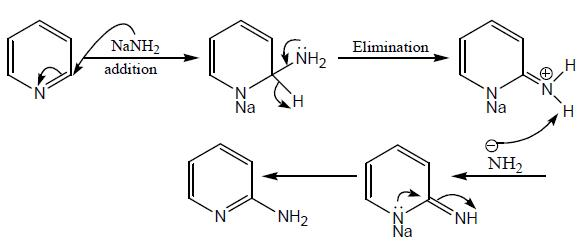QUESTION: 3

### The enzyme which cuts from the N-terminal side of the amino acids having Leu, Asp and Glu amino acids is/are

Solution:

Given, all the enzymes except pepsin cuts from the C-terminal side.

QUESTION: 4

The protecting group of amines which is deprotected by base is

Solution: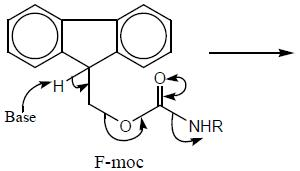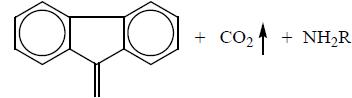*Answer can only contain numeric values
QUESTION: 5

The number of π-electrons which are in delocalization in pyridine ring is/are____

Solution: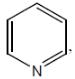there are three π-bonds in pyridine ring. So, that total of 6π-electrons are invoved in delocalization. Hence, aromatic.

*Answer can only contain numeric values
QUESTION: 6

Among the following sugars which gives both Bromine water and Tollen’s Test positive is/are
D-glucose, D-Fructose, D-mannose, D-iodose

Solution:

D-glucose, D-mannose and D-iodose all have aldehydic functional group and gives both positive tests.
While as D-fructose have ketonic functional group and gives negative bromine water test, but positive Tollen’s test.

*Answer can only contain numeric values
QUESTION: 7

The most reactive position for the nitration in the following compounds is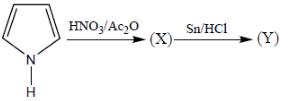Solution: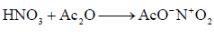(mild nitrating agent) electrophilic addition on pyrrol takes place at second position then third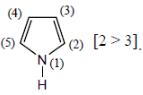And Sn/HCI converts NO2 to NH2 group.

*Answer can only contain numeric values
QUESTION: 8

If a tripeptide is treated with Sangers reagent followed by hydrolysis. How many amide bonds will be hydrolysed in a peptide chain?

Solution: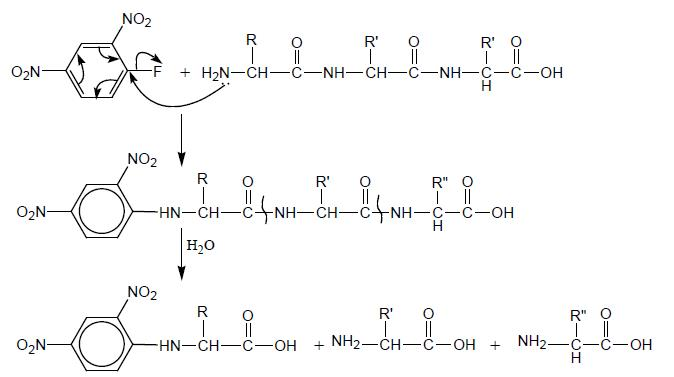*Answer can only contain numeric values
QUESTION: 9

In the following reaction, how many membered ring will be formed as major product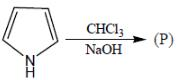Solution:

It is a Reimer-Tiemann reaction and ring expansion is a major product in this reaction,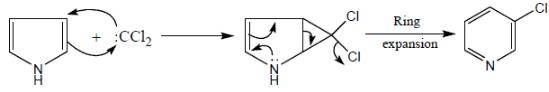*Answer can only contain numeric values
QUESTION: 10

Among the following amino acids, how many amino acids having a sulfur groups
(I) Cysteine   (II) Methionine  (III) Arginine  (IV) Valine  (V) Lyscine  (VI) Cystine

Solution:
QUESTION: 11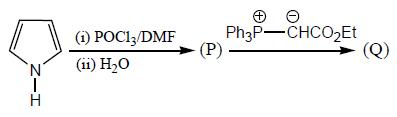Solution: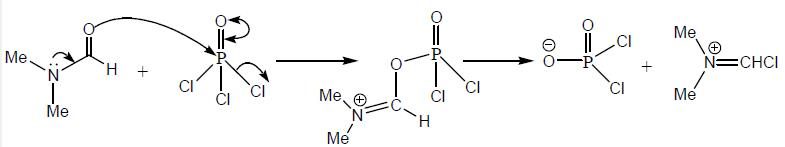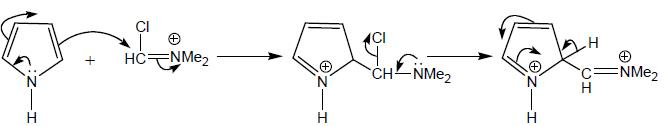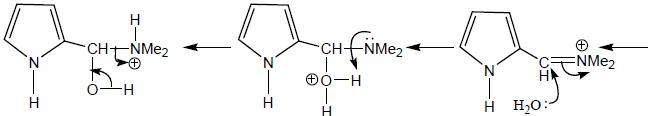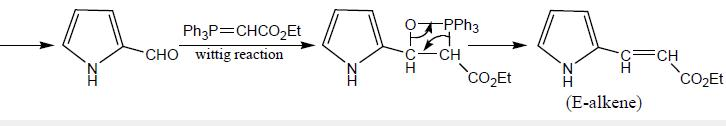QUESTION: 12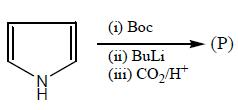Solution: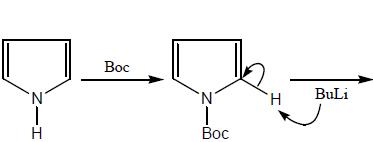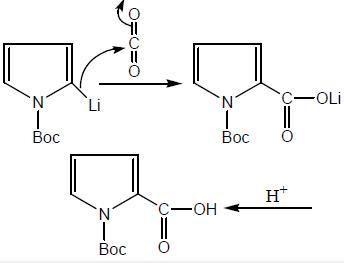QUESTION: 13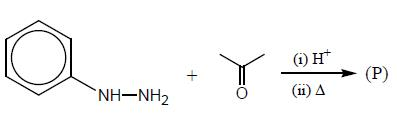Solution: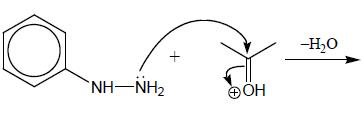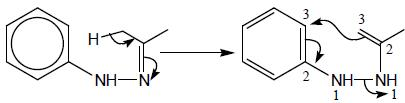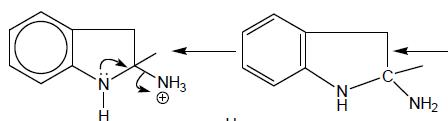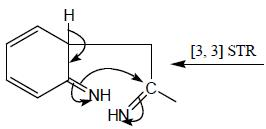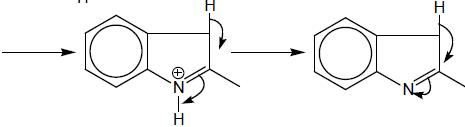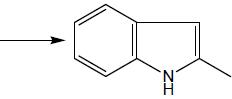QUESTION: 14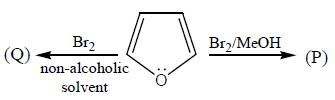Solution:

It is a solvent dependent reaction Brin presence of alcoholic solvent gives diacetal but in absence of alcoholic solvent gives polybromination product.

QUESTION: 15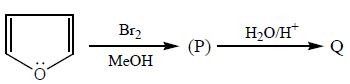Solution: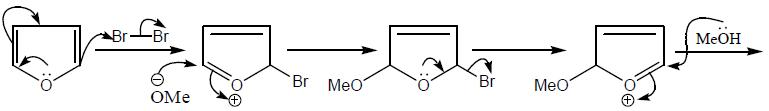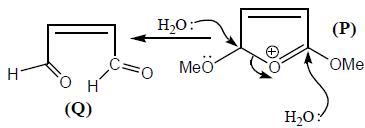QUESTION: 16

Give the aromaticity order in the following compounds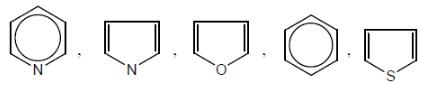Solution: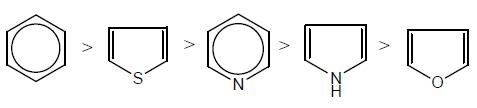QUESTION: 17

The products (A) and (B) formed in the following reaction sequence are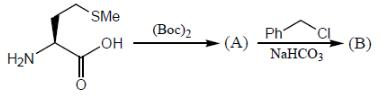Solution: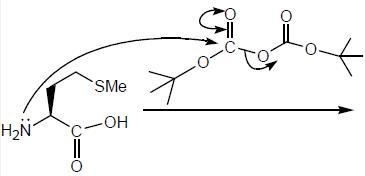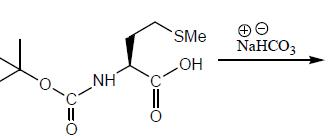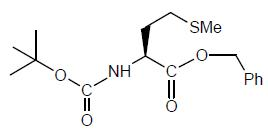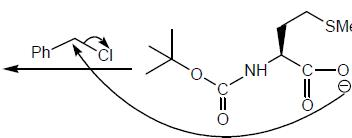QUESTION: 18

The osazone (A) could be obtained from :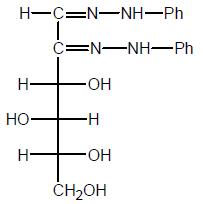Solution: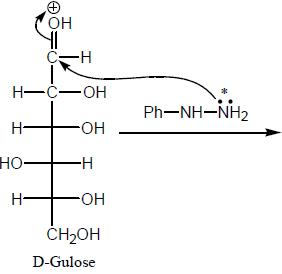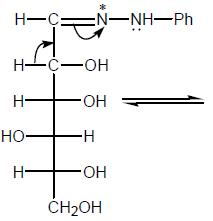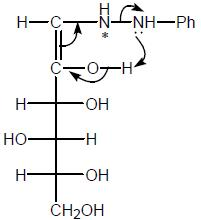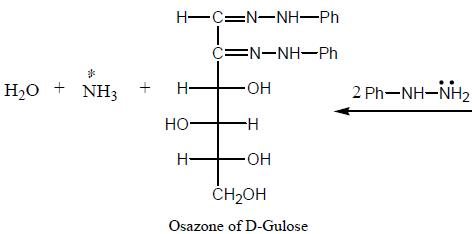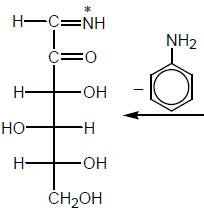Osazone of D-gulose and D-iodose.

*Answer can only contain numeric values
QUESTION: 19

Among the following amino acids, how many  is/are basic
Val, Arg, His, Cys, Leu, Lys, Met

Solution:

Arg, His, and Lys are the basic amino acids

*Answer can only contain numeric values
QUESTION: 20

Calculate the isoelectronic point value of the salt of Argnine, having pKa values; 10.9, 8.5, 2.2, respectively.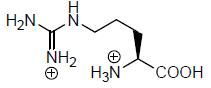Solution: